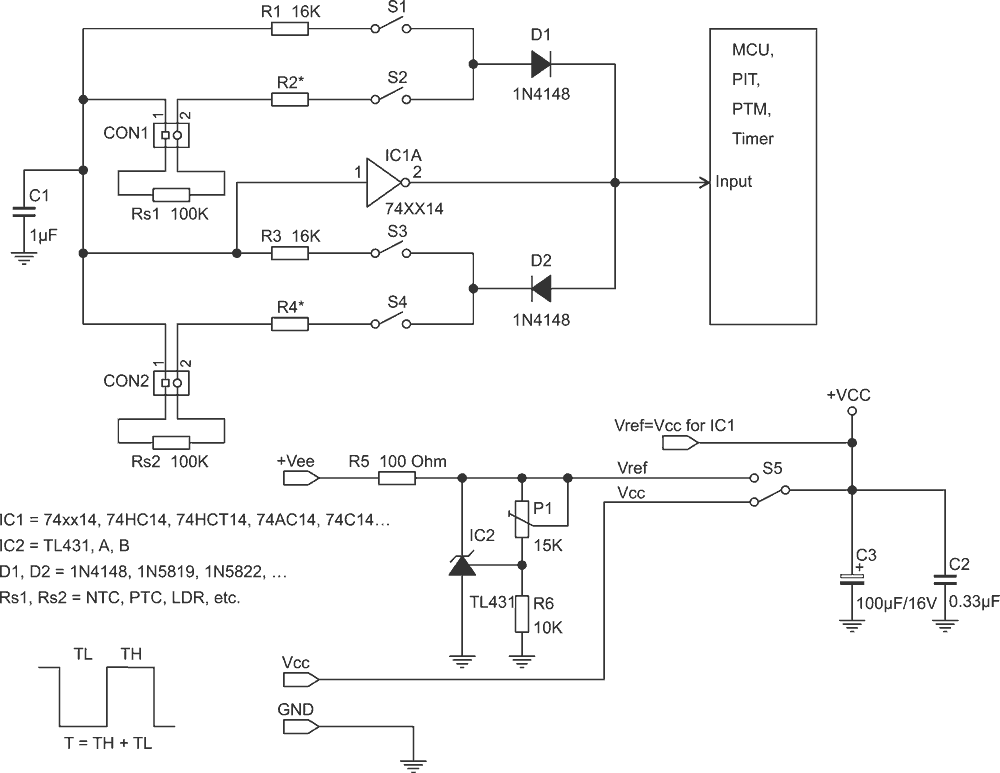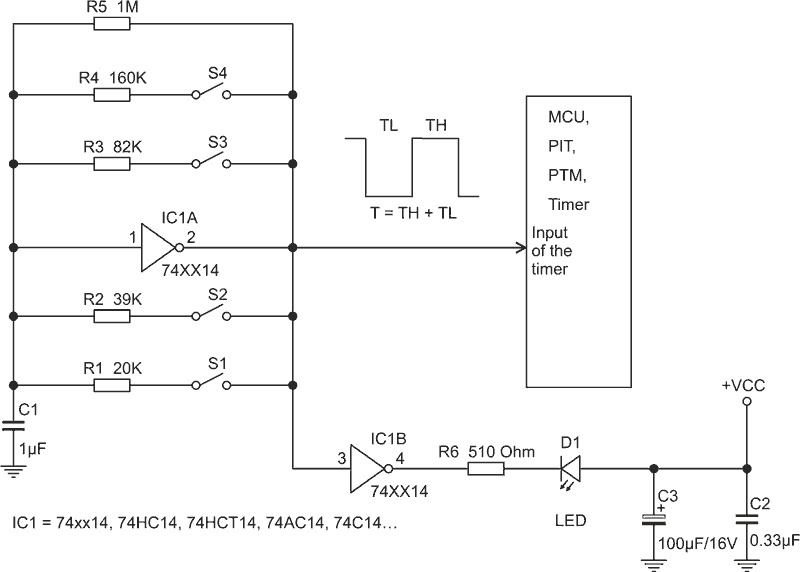# Measure two resistive sensors or multiple switches with a single Schmitt

Petre Petrov

EDN

Many of the sensors capturing information from the world around us are resistive. A few examples are NTC, PTC, LDR, & contact sensors. If we convert the resistance of the sensors into frequency or pulse duration, these parameters can be measured by most MCUs, without needing an ADC.Figure 1. Single inverter with Schmitt is serving two resistive sensors.

Figure 1 is showing how a single inverter with Schmitt trigger (74xx14 or 40106) is serving two resistive sensors, Rs1 and Rs2. One of the sensors is controlling the low time TL of the output pulses and the second sensor is controlling the high time TH. Diodes D1 and D2 make the high & low times independent of each other. Resistors R2 and R4 are not obligatory. They are used to offset the resistance of the sensors if needed.

R1 and R3 can be used if we want to alter the sensor response curve, or to provide threshold calibration points.

An example setup of the circuit follows, where R1 & R3 are used to calibrate set-points:

1. If the reference voltage Vref is needed for extra accuracy, S5 is set in the upper position, and the reference voltage Vref is set with trimmer potentiometer P1 to the required value, e.g. 5.0 V, 5.5 V, 4.5 V, etc.
2. The switches S1 and S3 are closed and the switches S2 and S4 are open. Now, R1 and R3 will determine the low and the high time of the output signal.
3. The low and high times are measured and stored in the memory of the MCU.
4. Now the switches S2 and S4 are closed and the switches S1 and S3 are open. In that position, the sensors Rs1 and Rs2 will determine the low and high times of the output signal.
5. The MCU measures the low and the high times and compares the measured values with the stored reference data.

The resistors R5, R6, and P1 around the reference source IC2 depend on the particular implementation and can be easily calculated with the formulas in the data sheet of the TL431A or equivalent IC.

Monitoring multiple contacts with a single inverter

A single inverter can also capture information from one or more contact sensors or switches. Figure 2 shows how a single Schmitt captures the status of four contacts, S1 to S4. The circuit converts the resistance in the feedback of IC1a into frequency. Diodes could also be added as in Figure 1 to increase the number of contacts.Figure 2. Single inverter with Schmitt is serving many contact sensors.

There are 16 possible combinations of open and closed switches. Each combination produces a square wave with predefined frequency. The frequencies can be measured and stored in the memory of the embedded MCU if calibration is necessary. R5 is used to maintain the oscillations when all switches are open. The resistors R1 to R5 can have any appropriate values. The important point is to have a different frequency for each combination of open and closed switches.

IC1b, R6, and D1 are not obligatory. They can make some of the combinations of open and closed switches visually recognizable if needed.

The number of the contact sensors is limited only by the resolution and the accuracy of the implementation. They depend on the IC, the stability of the power supply, the accuracy of the resistors in the feedback, and the capability of the MCU to measure the duration of the pulses and the frequency.

EDN